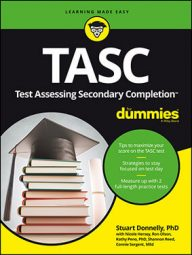##### TASC For DummiesYou will probably run into a quadratic question on the TASC Math exam. A quadratic is a specific type of polynomial equation that is of the form ax2 + bx + c = 0. One way to solve an equation in this form is through factoring.

Follow these steps to solve by factoring:

1. Be sure the equation is in standard form (all terms are on one side of the equal sign, the equation equals 0, and the terms are in descending order).
2. Identify a, b, and c.
3. Find the factors of ac that add together to give b.
4. Put each of these factors over a (the leading coefficient) and simplify if possible.
5. Set up two parentheses with the variable plus the simplified factors.
6. If the factors are in fraction form, move the denominator to be the coefficient of x.
7. Using the zero product property (which states that anything multiplied by zero equals zero), set each parenthesis equal to 0.
8. Solve each of the resulting equations.

It's important to note that not all quadratics are factorable. This doesn't mean there's no solution for the quadratic equation. It means that there may not be rational number answers, and your solution may have a radical or imaginary number as part of it.

Here are some example questions to try:

EXAMPLE 1

x2 + 5x + 6 = 0

a = 1 b = 5 c = 6

This means the two factors that multiply together to give 6 and add together to give 5 are 2 and 3.

This means the two solutions to this quadratic are –2 and –3.

EXAMPLE 2

6x2 + x – 2 = 0

a = 6 b = 1 c = –2

This means the two factors that multiply together to give –12 and add together to give 1 are –3 and 4.

This means the two solutions to this quadratic are 1/2 and –2/3.

Stuart Donnelly, PhD, was awarded a PhD in mathematics from Oxford University. He has prepared students for the TASC test and GED Test for the past two decades.

Stuart Donnelly, PhD, earned his doctorate in mathe-matics from Oxford University at the age of 25. Since then, he has established successful tutoring services in both Hong Kong and the United States and is considered by leading educators to be one of the most experienced and qualified private tutors in the country.

Stuart Donnelly, PhD, was awarded a PhD in mathematics from Oxford University. He has prepared students for the TASC test and GED Test for the past two decades.

Stuart Donnelly, PhD, was awarded a PhD in mathematics from Oxford University. He has prepared students for the TASC test and GED Test for the past two decades.

Sandra Luna McCune, PhD, is professor emeritus and a former Regents professor at Stephen F. Austin State University. She's now a full-time author. Shannon Reed, MA, MFA, is a visiting lecturer at the University of Pittsburgh, where she teaches composition, creative writing, and business writing.

Stuart Donnelly, PhD, was awarded a PhD in mathematics from Oxford University. He has prepared students for the TASC test and GED Test for the past two decades.